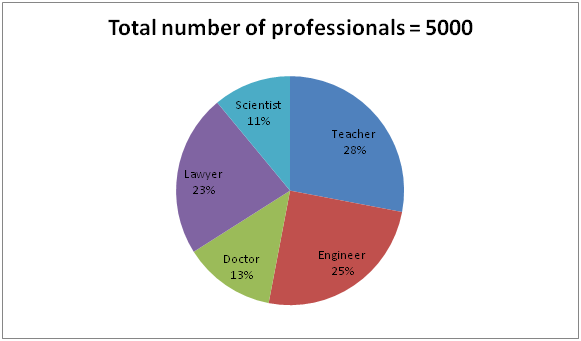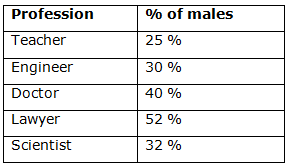# IBPS Clerk Prelims 2018 – Quantitative Aptitude Questions Day-8

Dear Readers, Bank Exam Race for the Year 2018 is already started, To enrich your preparation here we have providing new series of Practice Questions on Quantitative Aptitude – Section. Candidates those who are preparing for IBPS Clerk Prelims 2018 Exams can practice these questions daily and make your preparation effective.

[WpProQuiz 4040]

Directions (Q. 1 – 5) Study the following information carefully and answer the given questions.

The following pie chart shows the percentage distribution of total number of different professionals in a certain city.The table shows the percentage of male professionals among them.1) Total number of female teachers is approximately what percentage of total number of male doctors in a city?

a) 258 %

b) 312 %

c) 404 %

d) 450 %

e) 275 %

2) Find the ratio between the total number of male engineers to that of total number of female lawyers in a city?

a) 125: 184

b) 87: 134

c) 32: 59

d) 11: 23

e) None of these

3) Find the difference between the total number of teachers to that of scientist in a city?

a) 925

b) 775

c) 650

d) 850

e) None of these

4) Find the total number of female engineer, doctor and lawyer in a city?

a) 2589

b) 1817

c) 2345

d) 1692

e) None of these

5) Total number of female scientists is approximately what percentage more/less than the total number of male doctors in a city?

a) 58 % less

b) 36 % more

c) 44 % more

d) 44 % less

e) 58 % more

Directions (Q. 6 – 10) what approximate value should come in place of question mark (?) in the following questions?

6) (17.78)2 + 23 % of 1299 – (5/12) of 839 =? – (9.72)3

a) 850

b) 1700

c) 1275

d) 2135

e) 2450

7) 2153 + 627 = 28 % of 799 + (4/9) of (?)

a) 5750

b) 4320

c) 3800

d) 3370

e) 2850

8) 856 =? + 428 – (315 ÷ 4 × 8.74)

a) 1850

b) 2160

c) 2780

d) 2520

e) 1140

9) 713.41 – 263.78 ÷ (21.78 % of 201) =?

a) 825

b) 542

c) 636

d) 707

e) 913

10) 3174 =? – (4/7) of (43/16) of 2119

a) 7120

b) 6355

c) 5675

d) 4540

e) 3160

Direction (1-5) :

Total number of female teachers in a city

= > 5000*(28/100)*(75/100) = 1050

Total number of male doctors in a city

= > 5000*(13/100)*(40/100) = 260

Required % = [1050/260]*100 = 404 %

The total number of male engineers in a city

= > 5000*(25/100)*(30/100) = 375

The total number of female lawyers in a city

= > 5000*(23/100)*(48/100) = 552

Required ratio = 375: 552 = 125: 184

The total number of teachers in a city

= > 5000*(28/100) = 1400

The total number of scientist in a city

= > 5000*(11/100) = 550

Required difference = 1400 – 550 = 850

The total number of female engineer, doctor and lawyer in a city

= > [5000*(25/100)*(70/100)] + [5000*(13/100)*(60/100)] + [5000*(23/100)*(48/100)]

= > 875 + 390 + 552 = 1817

Total number of female scientists in a city

= > 5000*(11/100)*(68/100) = 374

Total number of male doctors in a city

= > 5000*(13/100)*(40/100) = 260

Required % = [(374 – 260)/260]*100 = 44 % more

Direction (6-10) :

(18)2 + 23 % of 1300 – (5/12) of 840 = x – (10)3

324 + (23/100)*1300 – (5/12)*840 = x – 1000

324 + 299 – 350 + 1000 = x

X = 1273 = 1275

2153 + 627 = 28 % of 799 + (4/9) of x

2780 = (28/100)*800 + (4/9)*x

2780 = 224 + (4x/9)

2556 = (4x/9)

X = 2556*(9/4) = 5751

X = 5750

856 = x + 428 – (316 ÷ 4 × 9)

856 = x + 428 – [(316/4)*9]

856 = x + 428 – 711

X = 856 – 428 + 711 = 1139

X = 1140

713.41 – 263.78 ÷ (21.78 % of 201) =?

713 – 264 ÷ [(22/100)*200] = x

X = 713 – 264/44

X = 713 – 6 = 707

3174 = x – (4/7) of (42/16) of 2120

3174 = x – (4/7)*(42/16)*2120

3174 = x – 3180

X = 3174 + 3180 = 6354 = 6355

Daily Practice Test Schedule | Good Luck

 Topic Daily Publishing Time Daily News Papers & Editorials 8.00 AM Current Affairs Quiz 9.00 AM Current Affairs Quiz (Hindi) 9.30 AM IBPS PO Mains- Reasoning 10.00 AM IBPS PO Mains– Quantitative Aptitude 11.00 PM Vocabulary (Based on The Hindu) 12.00 PM IBPS PO Mains– English Language 1.00 PM IBPS PO/Clerk – GK 3.00 PM Daily Current Affairs Updates 5.00 PM IBPS Clerk Prelims – Reasoning 6.00 PM IBPS Clerk Prelims – Reasoning (Hindi) 6.30 PM IBPS Clerk Prelims – Quantitative Aptitude 7.00 PM IBPS Clerk Prelims – Quantitative Aptitude (Hindi) 7.30 PM IBPS Clerk Prelims – English Language 8.00 PM